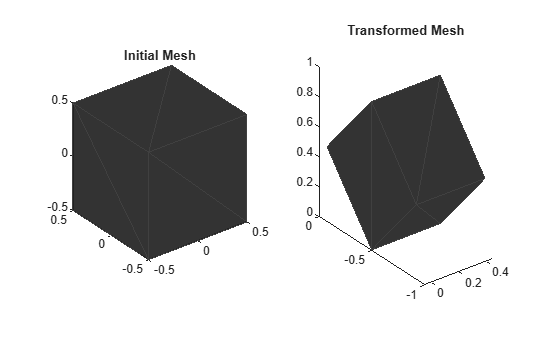applyTransform

Apply forward transformation to mesh vertices

Description

example

transformedMesh = applyTransform(mesh,T) applies the forward transformation matrix T to the vertices of the object mesh.

Examples

collapse all

Create an extendedObjectMesh object and transform the object by using a transformation matrix.

Create a cuboid mesh of unit dimensions.

cuboid = extendedObjectMesh('cuboid');

Create a transformation matrix that is a combination of a translation, a scaling, and a rotation.

tform = makehgtform('translate',[0.2 -0.5 0.5], ...
'scale',[0.5 0.6 0.7], ...
'xrotate',pi/4);

Transform the mesh.

transformedCuboid = applyTransform(cuboid,tform);

Visualize the meshes.

subplot(1,2,1);
show(cuboid);
title('Initial Mesh')

subplot(1,2,2);
show(transformedCuboid);
title('Transformed Mesh')Input Arguments

collapse all

Extended object mesh, specified as an extendedObjectMesh object.

Transformation matrix applied on the object mesh, specified as a 4-by-4 matrix. The 3-D coordinates of each point in the object mesh is transformed according to this formula:

[xT; yT; zT; 1] = T*[x; y; z; 1]

xT, yT, and zT are the transformed 3-D coordinates of the point.

Data Types: single | double

Output Arguments

collapse all

Transformed object mesh, returned as an extendedObjectMesh object.